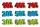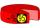# 3 years

The company has increased the number of employees. In year 2005 compared to 2004 13% more, in 2006 18% more against the previous year, in year 2007 20% more compared to 2006. How many % increase in the number of employees in 3 years?

Result

p =  60 %

#### Solution:Leave us a comment of example and its solution (i.e. if it is still somewhat unclear...):Be the first to comment!## Next similar examples:

1. EnrollmentThe enrollment at a local college increased 4% over last year's enrollment of 8548. Find the increase in enrollment (x1) and the current enrollment (x2).Calculate how many percent will increase the length of an HTML document, if any ASCII character unnecessarily encoded as hexadecimal HTML entity composed of six characters (ampersand, grid #, x, two hex digits and the semicolon). Ie. space as: &#x20;
3. Price increase 2xIf two consecutive times we increase the price of the product by 20%, how many % is higher final price than the original?
4. Double percentWhat is 80% of 60% of 2800?
5. Profit gainIf 5% more is gained by selling an article for Rs. 350 than by selling it for Rs. 340, the cost of the article is:
6. ShotsDetermine the percentage rate of keeper interventions if from 32 shots doesn't caught four shots.
7. Seeds 2How many seeds germinated from 1000 pcs, when 23% no emergence?
8. PersonsPersons surveyed:100 with result: Volleyball=15% Baseball=9% Sepak Takraw=8% Pingpong=8% Basketball=60% Find the average how many like Basketball and Volleyball. Please show your solution.
9. CancerOf the 80 people 50 people ill cancer. What percentage of people isn't ill?
10. IronIron ore contains 57% iron. How much ore is needed to produce 20 tons of iron?
11. PercentagesExpressed as a percentage:
12. Percentages52 is what percent of 93?
13. Percents - easyHow many percent is 432 out of 434?
14. ClassIn a class are 32 pupils. Of these are 8 boys. What percentage of girls are in the class?
15. Sales offGoods is worth € 70 and the price of goods fell two weeks in a row by 10%. How many % decreased overall?
16. ClassIn 7.C clss are 10 girls and 20 boys. Yesterday was missing 20% of girls and 50% boys. What percentage of students missing?
17. Base, percents, valueBase is 344084 which is 100 %. How many percent is 384177?